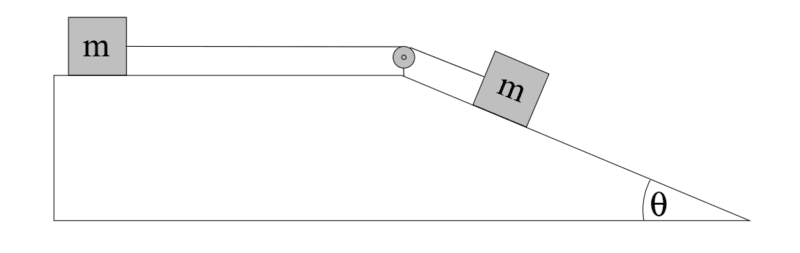# Will this system move?

## Homework Statement

two blocks of mass m sit on a frictionless surface and are connected by a massless, unstrechable string that rolls on a massless, frictionless pulley as shown. the second mass sits on an inclined tipped at an angle θ relative to horizontal. at time t=0, both masses are released from rest.
a) find the acceleration of both masses (magnitude, but indicate directions for each mass on the figure).
b) find the tension T in the string.Σf=ma

## The Attempt at a Solution

I can solve this one, but the only problem i am facing is that i see the whole system stationary. i can't indicate the direction of the acceleration and find the a of both masses. i just need to know will they move, and if yes how could that happen. i know that the incline will reduce the weight of the mass so the only force on the mass on the incline is mgsinθ, and this is even less from the first mass's weight.
or is it like since the floor is frictionless then the first mass will be moved and (so the system) by any Tension force even though it's very small. but what about the inertia then?

#### Attachments

.Scott
Homework Helper
The first mass is not being lifted. So the force to move it only needs to overcome friction - of which there is none.

•SakuRERE
The first mass is not being lifted. So the force to move it only needs to overcome friction - of which there is none.
then no friction means no resistance (inertia)?

i am sorry if i am asking basic questions, but for some reasons it's been a long time since i studied physics and i am trying to regain the flexibility of thinking that i used to have. however it appears that i am still lacking some basic information.

.Scott
Homework Helper
then no friction means no resistance (inertia)?
Inertia and resistance are two separate things.
There is no friction, so when you pull it it will start moving immediately.
But yes, there will be inertia. So the mass will begin to accelerate from stationary - gaining speed for as long as it is pulled - or until it hits that pulley.

•SakuRERE
Chestermiller
Mentor

## Homework Statement

two blocks of mass m sit on a frictionless surface and are connected by a massless, unstrechable string that rolls on a massless, frictionless pulley as shown. the second mass sits on an inclined tipped at an angle θ relative to horizontal. at time t=0, both masses are released from rest.
a) find the acceleration of both masses (magnitude, but indicate directions for each mass on the figure).
b) find the tension T in the string.
View attachment 231730

Σf=ma

## The Attempt at a Solution

I can solve this one, but the only problem i am facing is that i see the whole system stationary. i can't indicate the direction of the acceleration and find the a of both masses. i just need to know will they move, and if yes how could that happen. i know that the incline will reduce the weight of the mass so the only force on the mass on the incline is mgsinθ, and this is even less from the first mass's weight.
or is it like since the floor is frictionless then the first mass will be moved and (so the system) by any Tension force even though it's very small. but what about the inertia then?
Have you drawn free body diagrams for the two masses? If so, what are the force balance equations for the two masses?

•SakuRERE
CWatters
Homework Helper
Gold Member

## The Attempt at a Solution

I can solve this one, but the only problem i am facing is that i see the whole system stationary. i can't indicate the direction of the acceleration and find the a of both masses. i just need to know will they move, and if yes how could that happen.

Does it snow in your country? Have you ever been on a sledge? Rolled down s hill on a bike?

These are all examples of objects on a slope with no (or minimal) friction

i know that the incline will reduce the weight of the mass so the only force on the mass on the incline is mgsinθ, and this is even less from the first mass's weight.

Weight = mass * g

The angle of the slope doesn't change either mass or g.

or is it like since the floor is frictionless then the first mass will be moved and (so the system) by any Tension force even though it's very small. but what about the inertia then?

If there is a net force on an object it MUST accelerate. F=ma so a=F/m. The objects mass only effects how fast it accelerates.

Draw separate free body diagrams for each block.

I also recommend you try and build it using two toy cars (The wheels will help reduce friction) or even two ice cubes.

•SakuRERE
Chestermiller
Mentor
View attachment 231733 this is the fbd or the two masses
So, if you eliminate T from these two equations, what do you get?

•SakuRERE
So, if you eliminate T from these two equations, what do you get?
T = m a
+ mgsinθ - T= m a
________________________
mgsinθ = 2m a
and by eliminating m from both sides:
a= (g sinθ)/2
so, sir, you want me to conclude that the acceleration cannot be zero unless the θ itself is zero which can't be (since it's inclined). so there is definitely an acceleration! was it?

•